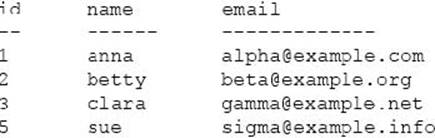# What is the outcome?

Consider the following table data and PHP code.

What is the outcome?

Table data (table name "users" with primary key "id"):PHP code (assume the PDO connection is correctly established):

\$dsn = ‘mysql:host=localhost;dbname=exam’;

\$pass=’********’;

\$pdo = new PDO{\$dsn, \$user, \$pass);

\$cmd ="SELECT* FROM users WHERE id= :id";

\$stmt = \$pdo->prepare(\$cmd);

\$id = 3;

\$stmt->bindParam(‘id’, \$id);

\$stmt->execute();

\$stmt->bindColumn{3, \$result);

\$row= \$stmt->fetch(PDO::FETCH_BOUND);
A . The database will return no rows.
B . The value of \$row will be an array.
C . The value of \$result will be empty.
D . The value of \$result will be ‘[email protected]’.